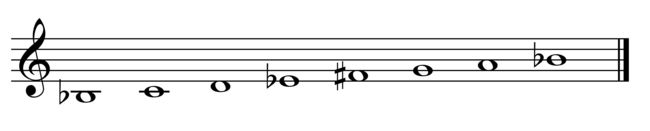# Lower The 6th Degree

Calculus Level 3$\large \frac{d^n}{dx^n} \frac{\ln(x)}{x} = \frac{a_n\ln(x)-b_n}{x^{n+1}}$

Let $f^{(n)}(x)$ be defined as the $n$-th derivative of $\frac{\ln(x)}{x}$.

If $f^{(n)}(x)$ can be written in the form shown above, then the solution to $f^{(n)}(x)=0$ can be written in the form $x=e^{\frac{p_n}{q_n}}$ where $p_n$ and $q_n$ are coprime positive integers.

What is $p_{10}+q_{10}$?

×